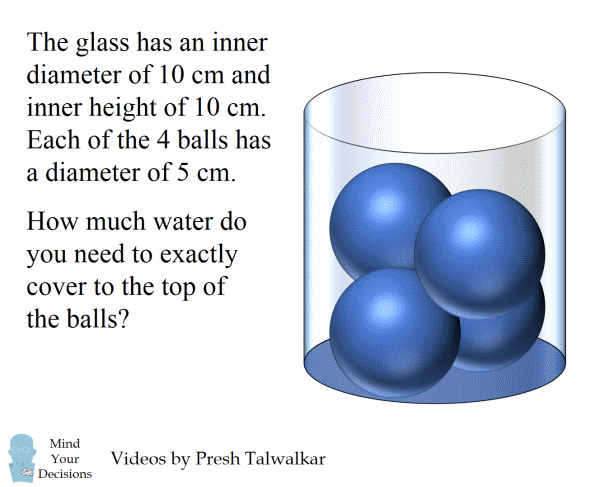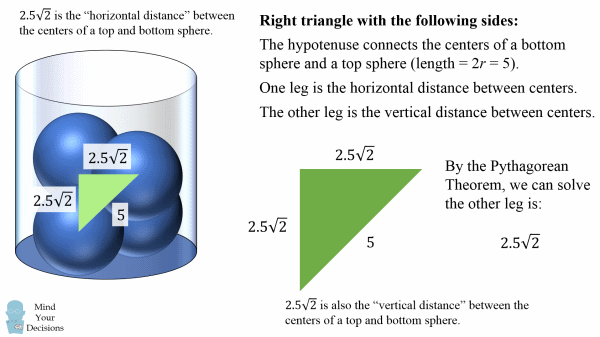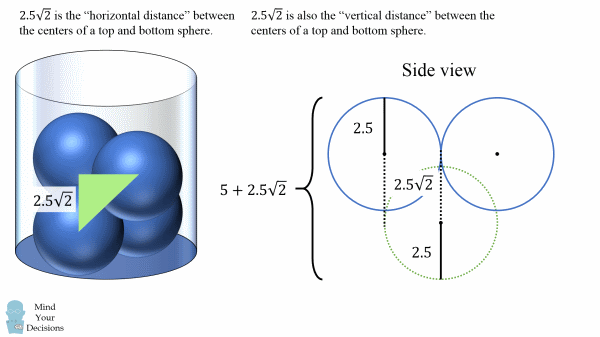The glass has an inner diameter of 10 cm and inner height of 10 cm. Each of the 4 balls has a diameter of 5 cm. How much water do you need to exactly cover to the top of the balls?You can assume the glass is a cylinder; the 4 balls are identical perfect spheres; and the top two balls are at a 90 degree angle to the bottom two balls.

I give a HUGE thanks to Stephen Lerantges for solving the problem. I got the wrong answer and was only able to see my mistake after reading his solution. I also thank Zack and Tomas for independently sending me this problem.

Can you solve it? Watch the video for a solution.

Can You Solve The Water Glass And 4 Balls Puzzle?

.
.
.
.
M
I
N
D
.
Y
O
U
R
.
D
E
C
I
S
I
O
N
S
.
P
U
Z
Z
L
E
.
.
.
.
Answer To The Water Glass And 4 Balls Puzzle

One way to approach the problem is to consider the top view. You will see the glass as a circle. Inside are circular borders for the top two spheres, which will be tangent to each other and the glass. The bottom two spheres will be a 90 degree rotation of the top two–so two circles that are tangent to each other and the glass.

The diameter of each sphere is 5 cm, so each radius is 2.5 cm. We can then solve the horizontal distance between the center of a top sphere and the center of a bottom sphere is 2.5√2 cm, as that is the hypotenuse of a right triangle where each leg is 2.5 cm.We can then consider a right triangle with the following sides.

Let the hypotenuse be the line segment connecting the centers of a top and bottom sphere. This hypotenuse will have a length of 2r = 5, as the circles are tangent to each other.

Let one leg be the horizontal distance, which we just derived was 2.5√2.

Let the other leg be the vertical distance. From the length of the other leg and hypotenuse, we can deduce we have an isosceles right triangle, so the vertical distance is also 2.5√2.Now we can look at the glass from the side. We want to figure out the height to the top of the balls. This will be the length of 2 radii plus the vertical distance between the centers, which is 2.5√2. So the total vertical distance is then 5 + 2.5√2.Now we can solve for the volume of water to reach the top of the balls. We consider the volume of a cylinder that reaches the top of the balls, and then we subtract the volume of 4 balls.The cylinder has a volume πR2h, where R = 5 and h = 5 + 2.5√2.

Each ball has a volume (4/3)πr3, where r = 2.5.

So the volume of water is:

volume(cylinder to top of balls) – volume(4 balls)

πR2h – (4/3)πr3

π(52)(5 + 2.5√2) – (4/3)π(2.5)3

125π(2 + 3√2)/6 � 408.580 cm3

So that’s the answer to this tricky problem!

Thanks to all patrons! Special thanks to:

Shrihari Puranik
Kyle

If you like my videos, you can support me at Patreon and get exclusive rewards: http://www.patreon.com/mindyourdecisions

Sources
I was shared an image that was credited to 9gag:
http://img-9gag-fun.9cache.com/photo/aVDZOEM_700b.jpg

This helped me see the “top view” solution
https://math.stackexchange.com/questions/2104548/spheres-in-cylinder-and-volume

Stephen actually found a solution in a slightly different way. Take 3 spheres and their centers form an equilateral triangle. You can then find the diagonal distance is the altitude, which can then be used to find the vertical distance between centers of spheres in two layers. Here is his write-up: